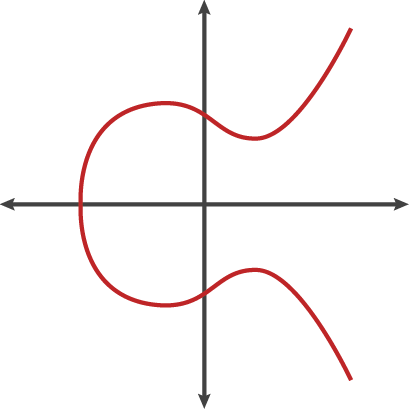# How to generate a public key from a private ECDSA key?

Having some specific ECDSA curve and a private key, how does one calculate the public key? I am having a hard time finding the algorithm and equations for it.

The write up on Wikipedia is pretty good. I won't go into all the detail that they do there, but your private key is a randomly selected integer $d_A$ selected from $[1,n-1]$ where $n$ is the order of the group. The public key is $Q_A=d_AG$ where $G$ is the base point on the curve defined in the publicly agreed upon parameters.

• Without defining your variables or the operations being performed, this answer is completely useless.
– B T
May 12, 2021 at 9:19
• @BT Answer seems pretty clear to me at at least the people who voted for it. May 12, 2021 at 21:47
• The only thing useful in your answer is the link to wikipedia. To understand anything else you wrote, you would need to already understand ECDSA cryptography and how eliptic curve math works.
– B T
May 14, 2021 at 3:03

I'm going to give a bad answer to this for the types of people like myself who aren't mathematicians, but who could reasonably solve FizzBuzz or calculate a Fibonacci sequence.

## TL;DR

You can't do this without a library (at least not as far as I can tell).

## A Mix of Terms

• d vs $$k$$, $$a$$, and $$b$$

d is the private key as JWK, but in the maths it's referred to as $$k$$ (or $$a$$ and $$b$$ if referring to private keys for each of Alice and Bob).

• x,y vs $$Q$$

Again, x and y are what we see in JWK, but formulas reference this as point $$Q$$ (or $$Q_a$$ and $$Q_b$$)

## Not as simple as $$Q_A=d_AG$$

Us layman see d(Gx, Gy) and we think that's easy!, you'd just get x = d * Gx and y = d * Gy.

If you multiply $$7$$ times the point $$(2, 4)$$ you get the point $$(14, 28)$$ - duh! Easy!

However, if you have a curve that looks like this...... well, if you just multiply a point by a number... you ain't gettin' a point on the curve. You're getting one way off in space somewhere.

In this way, the notation looks like, but isn't multiplication, per se. It's more like saying "do operation $$G$$, $$k$$ times". Yet you can't take the result of one iteration of $$G$$ and just multiply it, because, in essence, $$G(n + 1)$$ is dependent on $$G(n)$$ (kinda like a Fibonacci function, in a way).

You're kinda "walking" the curve as it were, where walking means... something about groups (starting at base group $$G$$) and doubling (both of which are specific mathematical terms), and wrapping at a specific max prime number (i.e. P-256, P-384, P-521).

That walk would take... eons, but there are some tricks that make it so that essentially whatever random number you pick is broken into factors of 2 and the number of operations, instead of being in the quintupa-quadrillions and taking months to perform, take only the sum of the factors to perform.

Say your number is $$585$$, then you'd have $$2^{9}+2^{6}+2^{3}+1$$ (meaning $$512+64+8+1$$), which would take 585 iterations of slow $$G$$, but only 19 iterations ($$9+6+3+1$$) of tricked-out fast $$G$$.

(hence, a person who knows the secret key can solve for $$Q$$ very quickly, because they know how it factors, but for someone who doesn't it'll take eons to solve)

This is also why ECDSA can be slower than RSA for signing and verification. Rather than a few operations on extremely large numbers, it's many operations on smaller numbers.

## Maths & Implementations

That was probably a bad explanation. It probably didn't convey nuance as deserved.

The best resource that I could find to explain how to get the Public Key from the Private key was this:

It's neither the oversimplified $$Q=dG$$, nor computer-optimized code. It's in the middle - about as high-level as it can be, still too complex for me to take in in a sitting.

And another:

And the simplest code samples I found were these: